# Principal fundamental solution

(diff) ← Older revision | Latest revision (diff) | Newer revision → (diff)

A fundamental solution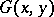, defined throughout the space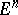, of a second-order elliptic equation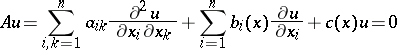(*)

that satisfies the conditionsfor certain positive constantsandif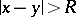.

If the coefficients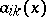,and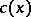satisfy a Hölder condition onand if the inequality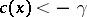is satisfied for some, then a principal fundamental solution exists. If the coefficients of the operatorare defined in a certain bounded domain with smooth boundary, then they can be extended to the entire spaceso that a principal fundamental solution will exist for the extended operator.

How to Cite This Entry:
Principal fundamental solution. Encyclopedia of Mathematics. URL: http://encyclopediaofmath.org/index.php?title=Principal_fundamental_solution&oldid=14964
This article was adapted from an original article by Sh.A. Alimov (originator), which appeared in Encyclopedia of Mathematics - ISBN 1402006098. See original article